# KSEEB Solutions for Class 8 Maths Chapter 14 Introduction of Graphs Ex 14.2

Students can Download Maths Chapter 14 Introduction of Graphs Ex 14.2 Questions and Answers, Notes Pdf, KSEEB Solutions for Class 8 Maths helps you to revise the complete Karnataka State Board Syllabus and score more marks in your examinations.

## Karnataka Board Class 8 Maths Chapter 14 Introduction of Graphs Ex 14.2

Question 1.
Draw the graphs of the following straight lines.
(i) y = 3 – x
(ii) y = x – 3
(iii) y = 3x – 2
(iv) y = 5 – 3x
(v) 4y = – x + 3
(vi) 3y = 4x + 1
(vii) x = 4
(vii) 3y = 1
(i) y = 3 – x

 x 0 1 2 y 3 2 1

When x = 0,                           y = 3 – 0 = (3)
When x = 1,                           y = 3 – 1 = (2)
Whenx = 2,                             y = 3 – 2 = (1)(ii) y = x – 3

 X 0 1 2 y -3 -2 -1

When x = 0,                           y = 0 – 3 = (- 3)
When x = 1,                        y = 1 – 3 = (- 2)
Whenx = 2,                            y = 2 – 3 = (- 1)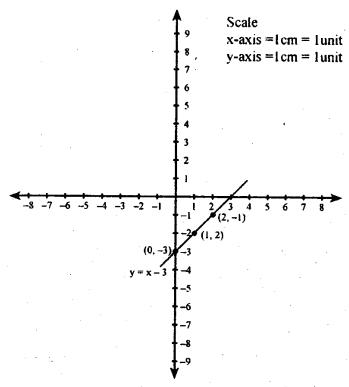(iii) y = 3x – 2

 X 0 1 2 y -2 1 4

When x = 0,                           y = 3(0) – 2 = (- 2)
When x = 1,                        y = 3(1) – 2 = (1)
Whenx = 2,                            y =3(2) – 2 = (4)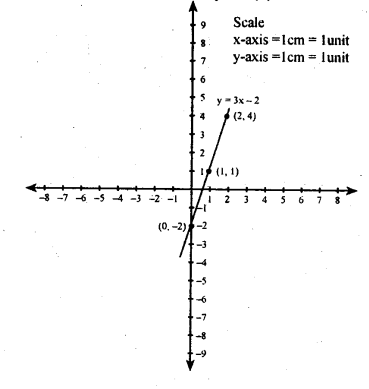(iv) y = 5 – 3x

 X 0 1 2 y 5 2 -1

When x = 0,                y = 5 – 3(0) = 5
When x = 1,                y = 5 – 3(1) = 2
Whenx = 2,                y = 5 – 3(2) = 5 – 6 = -1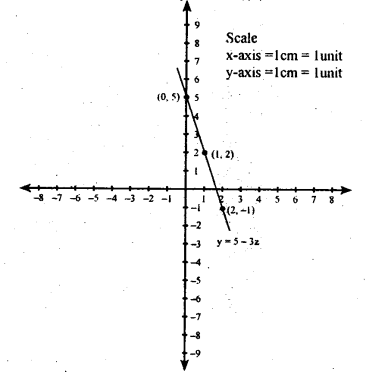(v) 4y = – x + 3(vi) 3y = 4x + 1

 X -1 2 5 8 y -1 3 7 11 (x,y) (-1,0 (2,3) (5,7) (7,11)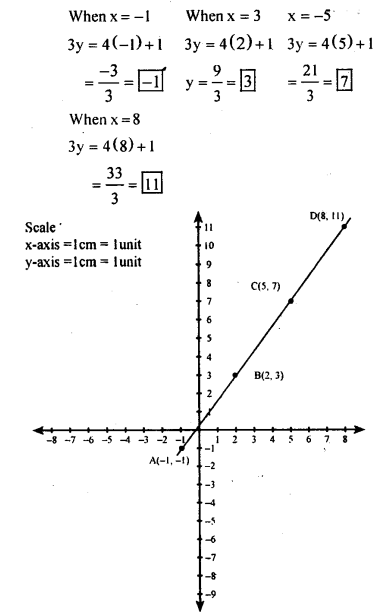(vii) x = 4

 X 4 4 4 4 y 0 1 2 3 (x,y) (4,0) (4,0 (4,2) (4,3)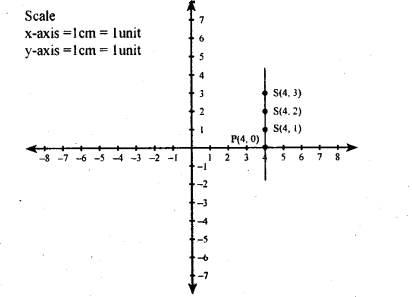(vii) 3y = 1Question 2.
Draw the graph of $$\frac{y}{x}=\frac{y+1}{x+2}$$
When x = 1Question 3.
Determine the equation of the line in each of the following graphs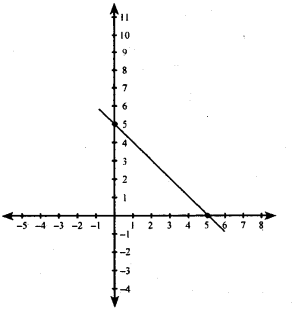(i) Let the equation be y = ax + b
We find that the lines passes through (2,0)
i. e., when x = 2, y = 0
∴ y = ax+b
0 = ax + b
0 = a(2) + b
0 = 2a + b
∴ b = – 2a
The line passes through (4,3) also
i. e., when x=4 , y = 3
∴ y = ax + b
3 = a(4) + b
3 = 4a + b
3 = 4a + (-2a)
3 = 4a = 2a
a = $$\frac { 3 }{ 2 }$$ b = – 2a = $$-2 \cdot \frac{3}{2}$$ = -3
y = ax + b y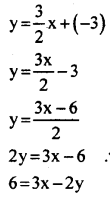The equation is 3x – 2y = 6

(ii) Let the equation be y = ax + b
we find that the line passes through (5,0) i.e., when x = 5, y = 0
∴ y = ax + b
0 = a(5) + b
0 = 5a + b
b = -5a
The line passes through (0 , 5)also
i.e.,when x = 0, y = 5
y = ax + b
5 = a(0) + b
5 = 0 + b
5 = 0 + (-5a)
5 = -5a
∴ a =$\frac { -5 }{ 5 }$
a = -1
b = -5a = -5(-1) = 5 b = 5
b = 5
y=ax + b
y = – 1 x + 5 y = – x + 5 y + x = 5
The equation of the line of is y + x = 6

Question 4.
A boat is moving in a river, downstream, whose stream has speed 8km/ hr. the speed of the motor of the boat is 22km/hr. Draw the graph of the distance covered by the boat versus an hour.
Speed of the boat downstream = 22 + 8 = 30 km/hr
y = 30x

 Time i 2 3 4 5 Distance 30 60 90 120 150 (1,30) (2,60) (3,90) (4.120) (5,150)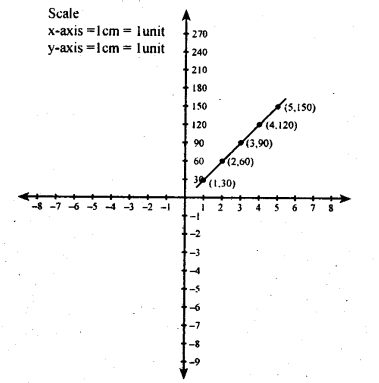Question 5.
Find the point of intersection of the straight lines 3y + 4x = 7 and 4y + 3x = 7, by drawing their graphs and looking for the point where they meet.
3y + 4x = 7
3y = 7 -4x
$$y-\frac{7-4 x}{3}$$

 X l 4 y 1 -3 (x,y) (1 × 1) (4-3)

When x=1, $$y-\frac{7-4 x}{3}$$ = $$\frac{7-4}{3}=\frac{3}{3}=1$$
When x = 4,X -1 5 y 2.5 -2 . (x,y) (-1,2.5) (5-2)error: Content is protected !!